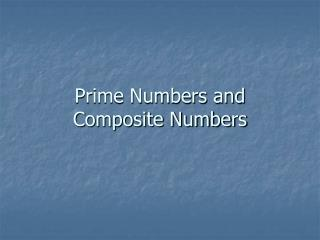# Prime Numbers and Composite Numbers - PowerPoint PPT PresentationDownload PresentationPrime Numbers and Composite Numbers

Prime Numbers and Composite NumbersDownload Presentation## Prime Numbers and Composite Numbers

- - - - - - - - - - - - - - - - - - - - - - - - - - - E N D - - - - - - - - - - - - - - - - - - - - - - - - - - -
##### Presentation Transcript

1. Prime Numbers and Composite Numbers

2. Some whole numbers can be divided up evenly, and some can't! A number that can not be divided up evenly is a Prime Number Example: 7 cannot be divided up evenly: Example: 6 can be divided evenly by 2, or by 3: 6 = 2 × 3 A number that can be divided up evenly is a Composite Number or divided into 2 groups divided into 3 groups So 6 is Composite, but 7 is Prime.

3. What is a Prime Number ? • Example: is 7 a Prime Number or Composite Number? • We can of course divide 7 into seven 1s (or one 7) like this: • 7 = 1 x 7 • But we could do that for any whole number! So we should also say we are not interested in dividing by 1, or by the number itself. • You cannot divide 7 evenly by 2 (you would get 2 lots of 3, with one left over) • You cannot divide 7 evenly by 3 (you would get 3 lots of 2, with one left over) • You cannot divide 7 evenly by 4, or 5, or 6. A Prime Number cannot be divided evenly by any number, except 1 or itself. So 7 is a Prime Number

4. What is a Composite Number? A Composite Number can be divide evenly by numbers other than 1 or itself. • Example: is 12 a Prime Number or Composite Number? • You can divide 12 evenly by 1 and 12. • 1 × 12 = 12 • You can divide 12 evenly by 2 and 6 (you would get 2 lots of 6 or 6 lots of 2). • 2 × 6 = 12 • You can divide 12 evenly by 3 and 4 (you would get 3 lots of 4 or 4 lots of 3). • 3 × 4 = 12 • We can divide 12 evenly by 1, 2, 3, 4, 6 and 12: So 12 is also a Composite Number

5. Important points to remember • Any whole number greater than 1 is either a Prime number or a Composite number. • 1 is considered neither a prime number nor a composite number • Remember this is only about Whole Numbers (1, 2, 3, ... etc), not fractions or negative numbers. • So don't say "I could multiply ½ times 6 to get 3“.

6. Examples From 1 to 14

7. Why All the Fuss about Prime and Composite? Because you can "break apart" Composite Numbers into Prime Number factors. It is like the Prime Numbers are the basic building blocks of all numbers. And the Composite Numbers are made up of Prime Numbers multiplied together. And that is why they are called "Composite" Numbers because composite means "something made by combining things“

8. This idea is so important it is called “The Fundamental Theorem of Arithmetic.”